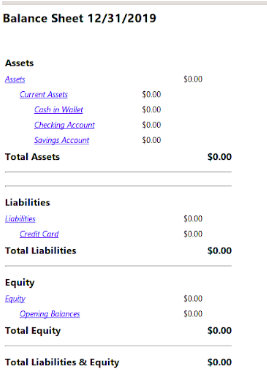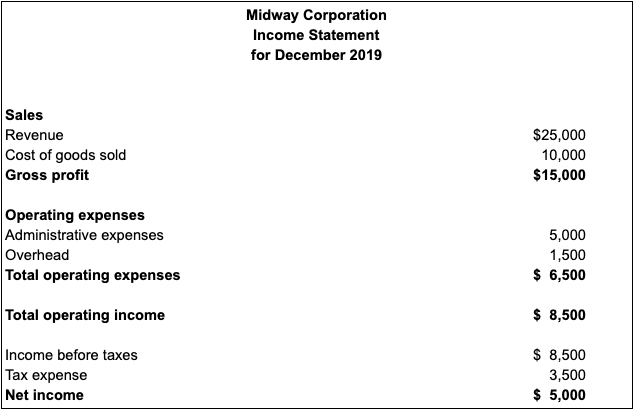Updated December 19, 2019

If you’re routinely performing all of the necessary bookkeeping basics for your business, why not start calculating basic accounting ratios?

Accounting ratios are calculated using the numbers found on your balance sheet and income statement, and they are designed to provide you with vital information that can help you better manage your business, both now and in the future.

## Overview: What are accounting ratios?

While they may initially appear complicated, accounting ratios are simple calculations that use the numbers found on your company’s financial statements. Accounting ratios can be performed using a simple calculation, and some accounting software applications even calculate these ratios in their reporting modules.

These simple ratios can be used to calculate projected growth and compare performance (such as between multiple store locations), and they can be of tremendous help when seeking funding from investors or financial institutions.

While ratio analysis can be complex, and a thorough job may be better suited to your CPA, performing basic accounting ratios is a simple process any business owner can do.

### Balance sheet vs. income statement: What’s the difference?

Your financial statements are designed to provide you with an in-depth analysis of your business. But these reports, such as a balance sheet and an income statement, are also needed in order to calculate accounting ratios.

While both are valuable resources for creating ratios, they contain very different information.

A balance sheet displays company assets, liabilities, and equity totals for a specific time. For instance, if you run a balance sheet dated December 31, the balance sheet will display total assets, liabilities, and equity as of December 31. See the example from Gnucash below:This GnuCash balance sheet displays assets, liabilities, and equity totals for a specific date.

All balance sheets must use the following formula:

Total assets = Total liabilities + Equity

A balance sheet can provide investors and financial institutions with a complete snapshot of a company’s financial health as of any specific date.

On the other hand, an income statement provides details on a company’s revenue and expenses for a specific period of time, such as the entire month of December, the entire quarter, or the entire year.

If you choose to run an income statement for December, you will be provided with information on revenue and expenses the company incurred or earned only during the month of December.This income statement reflects all revenue and expenses for only the month of December.

An income statement is one of the best ways to determine if a business is making a profit or losing money. It also compares performance, so you can run an income statement for December of 2019 and an income statement for December of 2018, and compare the performances for each year.

## The best accounting ratios for small businesses to track

Accounting ratios range from simple to complex. As a small business owner, you likely want to focus on the simpler ratios that are designed to provide valuable information about your business and its financial health.

Here are a few accounting ratios you can easily calculate that provide you with the information you need.

### 1. Gross profit margin ratio

Your gross profit margin is one of the most important ratios you can calculate for your business, and doing so is easy.

Using numbers from your income statement, take your total revenue and subtract cost of goods sold. That total is then divided by revenue to obtain your gross profit margin.

For example, if your revenue is \$25,000 and your cost of goods sold is \$10,000, your total net revenue is \$15,000. You would then divide your net revenue by your gross revenue to obtain your gross profit margin ratio. The formula looks like this:

Gross profit ratio = (Revenue - Cost of goods sold) / Revenue

Using the numbers above, the calculation would be:

\$25,000 - \$10,000 / \$25,000 = 0.60

That means you have a gross profit margin of 60%, earning \$0.60 for each dollar you receive in sales.

### 2. Profit margin ratio

Similar to the gross profit margin ratio, the profit margin ratio lets you know exactly how much you earn after subtracting all expenses, not just cost of goods sold.

This allows you to see exactly how much profit you make after all expenses are paid, giving you a good indication of how well your business is performing overall.

The formula for calculating your profit margin is:

Profit margin = (Revenue - Expenses) / Revenue

\$25,000 - \$18,500 = \$6,500 / \$25,000 = 0.026

A good indicator of a healthy business is a 25% profit margin or higher.

### 3. Quick ratio

A quick ratio can be used to evaluate the ability of your business to meet its immediate financial obligations. Quick ratio numbers are obtained from your balance sheet and compare your asset total with your liability total.

The formula to calculate a quick ratio is:

Quick ratio = (Current assets - Inventory) / Current liabilities

If your company has current assets of \$90,000 and inventory of \$30,000, your current asset total would be \$60,000. You would then divide that number by the amount of your current liabilities, which are \$35,000, to obtain your quick ratio.

\$90,000 - \$30,000 = \$60,000 / \$35,000 = 1.71

This means you currently have \$1.71 in assets for each \$1.00 in liabilities. Businesses should have at least a one-to-one ratio, meaning you’d have as much (or more) in assets than liabilities.

### 4. Current ratio

Like the quick ratio, the current ratio uses your asset and liability totals, but in this case, they are used to determine the ability of your company to pay long-term debt.

The only difference in the calculation is the inclusion of any inventory totals. If you do not have inventory, a current ratio calculation and a quick ratio calculation would have the same result.

A current ratio formula is:

Current ratio = Current assets / Current liabilities

Using the numbers from the previous example, your current ratio would be:

\$90,000 / \$35,000 = 2.57

This means for every \$1 in liabilities, you have \$2.57 in assets.

### 5. Debt-to-equity ratio

A debt-to-equity ratio, sometimes known as a debt-to-asset ratio, displays the ratio of company liabilities to shareholder equity. This is a good indicator of how much of your business assets are financed by debt such as loans, and how much are financed by owner equity.

Calculating a debt-to-equity ratio is fairly simple and uses numbers from your balance sheet:

Debt-to-equity ratio = Total liabilities / Shareholder’s equity

A business with \$125,000 in liabilities and \$180,000 in shareholder’s equity would have a debt-to-equity ratio of 0.69.

\$125,000 / \$180,000 = 0.69

Any value higher than 1 means more of your company’s assets are financed by debt, something to avoid if possible.

### 6. Return on investment (ROI) ratio

A return on investment ratio allows you to see how much of your original investment into your business has turned into profit. This is an excellent ratio for newer businesses, allowing you as the initial investor to see just how much (or little) your original investment has paid off.

The formula for calculating ROI is simple:

ROI = (Earnings - Investment) / Investment